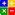# Ratio - Inventory Turnover RatioINVENTORY TURNOVER RATIO:Online Inventory Turnover Ratio Calculator

This ratio is obtained by dividing the 'Total Sales' of a company by its 'Total Inventory'. The ratio is regarded as a test of Efficiency and indicates the rapiditity with which the company is able to move its merchandise.

The formula:

Inventory Turnover Ratio = Net Sales / Inventory

The formula:

This ratio can also be calculated as:

Inventory Turnover Ratio = Cost of Goods Sold / Inventory